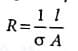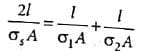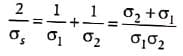Two metal wires of identical dimensions are connected in series. If σ1 and σ2 are the conductivities of the metal wires respectively, the effective conductivity of the combination is
• a)
σ + σ2
• b)
σ + σ2 / 2
• c)
√σ1σ2
• d)
1σ/ σ + σ2

### Related TestNew Words Jan 30, 2022Resistance of a wire in terms of connectivity (σ) is given bywhere l is the length and A is area of cross section of wire respectively.
∴ Rs = R1 + R2
⇒where σs is the effective conductivityσs = 1σ/ σ + σ2

Resistance of a wire in terms of connectivity (σ) is given bywhere l is the length and A is area of cross section of wirerespectively.∴ Rs = R1 + R2⇒where σs is the effective conductivityσs =2σ1σ2/σ1+σ2
Do you know? How Toppers prepare for NEET Exam
With help of the best NEET teachers & toppers, We have prepared a guide for student who are preparing for NEET : 15 Steps to clear NEET Exam